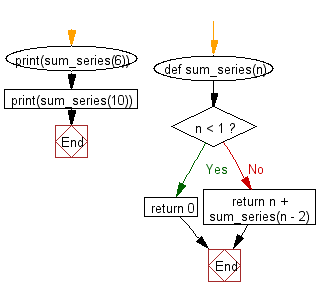﻿ Python Data Structures and Algorithms - Recursion: Sum of n+(n-2)+(n-4)... - w3resource# Python Data Structures and Algorithms - Recursion: Sum of n+(n-2)+(n-4)...

## Python Recursion : Exercise-7 with Solution

Write a Python program to calculate the sum of the positive integers of n+(n-2)+(n-4)... (until n-x =< 0).

Sample Solution:

Python Code:

``````def sum_series(n):
if n < 1:
return 0
else:
return n + sum_series(n - 2)

print(sum_series(6))
print(sum_series(10))
```
```

Sample Output:

```12
30
```

Flowchart:## Visualize Python code execution:

The following tool visualize what the computer is doing step-by-step as it executes the said program:

Python Code Editor:

What is the difficulty level of this exercise?

Test your Programming skills with w3resource's quiz.

﻿

## Python: Tips of the Day

Decapitalizes the first letter of a string:

Example:

```def tips_decapitalize(s, upper_rest=False):
return s[:1].lower() + (s[1:].upper() if upper_rest else s[1:])
print(tips_decapitalize('PythonTips'))
print(tips_decapitalize('PythonTips', True))
```

Output:

```pythonTips
pYTHONTIPS
```